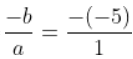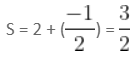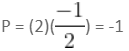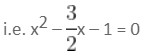Which of the following statements regarding ...
Which of the following statements regarding quadratic equations is/are true?
(A) The quadratic equation 2x2 + ax + a = 0 has real and equal roots and the values of a are 0 and 8.
(B) The sum and the product of the roots of the equation x2 - 5x + 6 = 0 are 5 and 6, respectively.
(C) The roots 2 and - 1/2 form the equation 2x2 - 3x - 2 = 0.
• a)
(A), (B) and (C)
• b)
(A) and (B)
• c)
(B) and (C)
• d)
(C) and (A)
• e)
Only (A)
Which of the following statements regarding quadratic equations is/ar...
For (A)
For equal roots, the discriminant D of 2x2 + ax + a = 0 should be zero.
D = a2 – 4(2)(a) = 0
a2 – 8a = 0
a(a – 8) = 0
a = 0, 8.
So, (A) is correct.
For (B)
Comparing x2 - 5x + 6 = 0 and ax2 + bx + c = 0,
a = 1, b = - 5 and c = 6
Therefore,
Sum of the roots == 5
Product of the roots = c/α = 6/1 = 6
For (C)
The given roots are 2 and -1/2.
Therefore, sum of the roots,And product of the given roots,Therefore, the required equation is:
x2 – Sx + P, i.e. x2 - (sum of the roots) x + product of the roots = 0i.e. 2x2 - 3x - 2 = 0
 1 Crore+ students have signed up on EduRev. Have you?
1 Crore+ students have signed up on EduRev. Have you?

### Learn this topic in detailCMAT Mock Test - 6 (New Pattern) 100 Ques | 180 MinsMore from Related Course Quantitative Aptitude (Quant)View courses related to this question

### Quick links for CAT exam850+
Video Lectures2500+
Revision Notes600+
Online Tests34+
Courses
Which of the following statements regarding quadratic equations is/are true?(A) The quadratic equation 2x2 + ax + a = 0 has real and equal roots and the values of a are 0 and 8.(B) The sum and the product of the roots of the equation x2 - 5x + 6 = 0 are 5 and 6, respectively.(C) The roots 2 and - 1/2 form the equation 2x2 - 3x - 2 = 0.a)(A), (B) and (C)b)(A) and (B)c)(B) and (C)d)(C) and (A)e)Only (A)Correct answer is option 'A'. Can you explain this answer?
Question DescriptionExplore Courses for CAT exam